##### Actions

(diff) ← Older revision | Latest revision (diff) | Newer revision → (diff)

A new method for estimating trigonometric sums (see Trigonometric sums, method of). By Vinogradov's method one may obtain very accurate estimates of a wide class of trigonometric sums, in which the summation variable runs through a sequence of integers, prime numbers, etc. In this way many classical problems in analytic number theory can be solved (the distribution of the fractional parts of a wide class of functions, the distribution of prime numbers in series of natural numbers, additive problems such as the Waring and Goldbach problems, etc.).

One distinguishes two parts in Vinogradov's method: The method of estimating Weyl sums (cf. Weyl sum) and the method of estimating trigonometric sums with prime numbers. Both these parts utilize Vinogradov's basic idea — to wit, the idea of smoothing double trigonometric sums, which may be described as follows. Given the sum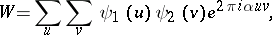where the summation variablesandrun through the values of (not necessarily successive) integers in respective setsand,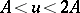. Letandbe arbitrary complex-valued functions. Thenwhereruns through the successive integers in the interval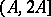(smoothing), and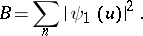## Vinogradov's method for estimating Weyl sums.

The sums to be estimated are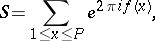where; here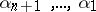are real numbers. For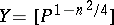one finds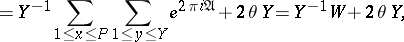where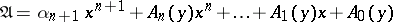, the letterdenotes the double sum overand, and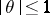. Moreover, letting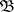denote the expression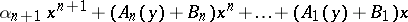one has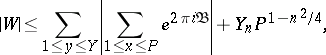for arbitrary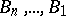from the domain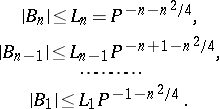For any integer: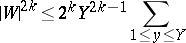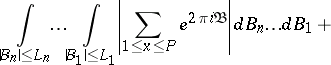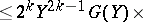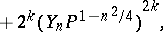whereandis the maximum number of cases of coincidence of points with coordinatesHere the braces denote the fractional part of the enclosed number, whilevaries between 1 and, and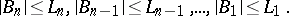If the coefficients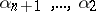of the polynomialhave certain arithmetical properties, it is possible to obtain the estimate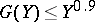. In addition, the last integral does not exceed the number of solutions of the system of equations:The number of solutions of this system is estimated using the Vinogradov theorem about the average, which is fundamental in Vinogradov's method for estimating Weyl sums (cf. Vinogradov estimates).

## Vinogradov's method for estimating trigonometric sums with prime numbers.

The sums to be estimated are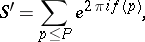where, and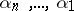are real numbers. Let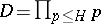, where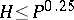. Using the well-known property of the Möbius function,is reduced to a small number of sums (this number is not larger than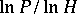) of the form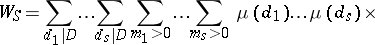where. In the multiple sum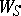the variables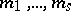run through the entire summation intervals. The sumsin which the summation interval over at least one variableis long are estimated by Vinogradov's method for estimating Weyl sums. Otherwise the summation interval over one of the summation variables,, will be long. In such a case one uses the following lemma of Vinogradov which, together with the idea of smoothing double sums, is fundamental to Vinogradov's method for estimating trigonometric sums with prime numbers.

Lemma. Letand letbe the product of all primes not larger than; all divisorsofnot larger thanmay then be distributed over fewer thansets with the following properties:

1) the numbersbelonging to one of these sets have the same numberof prime factors and therefore the same value of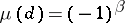;

2) one of these sets — the so-called simplest set — consists of the single number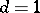. For each of the remaining sets there is a numbersuch that all numbers of this set satisfy the relation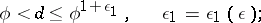3) for each set of numbersother than the simplest set there exist, for any,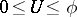, two sets of numbers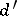and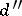, with corresponding numbers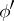andsatisfying the relations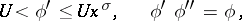and such that, for a certain natural number, one obtains each number in the chosen settimes if, out of all the productsone selects only those which satisfy the relation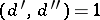.

The application of point 3) of this lemma, with a suitable value of, yields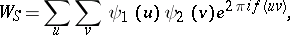where the variablesandrun through long summation intervals. It is possible to deduce Vinogradov's estimate for trigonometric sums with prime numbers from this lemma (cf. Vinogradov estimates).

If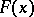can be properly approximated, in a certain sense, by a polynomial, Vinogradov's method can be used to estimate sums of the type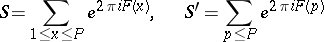(see , ). Sums of the type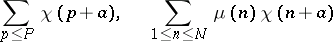and other types can also be estimated by the method. It is possible in this way to solve problems on the distribution of power residues, primitive roots, etc., in sequences of the type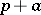, whereis a given integer, whileruns through the successive prime numbers , . For the application of Vinogradov's method in analytic number theory see , , , , .

How to Cite This Entry: